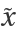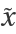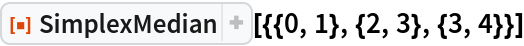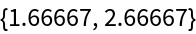Function Repository Resource:

# SimplexMedian

Get the simplex median of the elements of a matrix

Contributed by: Wolfram Research
 ResourceFunction["SimplexMedian"][matrix] gives a simplex median of the elements in matrix.

## Details and Options

ResourceFunction["SimplexMedian"][matrix] gives a vectorminimizing the sum of the volumes of the p-dimensional simplices formed by the elements of matrix and.

## Examples

### Basic Examples (1)

Simplex median for bivariate data:

 In:=Out=## Requirements

Wolfram Language 11.3 (March 2018) or above

## Version History

• 1.0.0 – 06 February 2019